Win up to 100% scholarship on Aakash BYJU'S JEE/NEET courses with ABNAT Win up to 100% scholarship on Aakash BYJU'S JEE/NEET courses with ABNAT

# JEE Advanced Question Paper 2022 Chemistry Paper 2

## JEE Advanced 2022 Chemistry Question Paper 2 with Solutions

SECTION – 1 (Maximum marks : 24)

∙ This section contains EIGHT (08) questions.

∙ The answer to each question is a SINGLE DIGIT INTEGER ranging from 0 TO 9, BOTH INCLUSIVE.

∙ For each question, enter the correct integer corresponding to the answer using the mouse and the on-screen virtual numeric keypad in the place designated to enter the answer.

∙ Answer to each question will be evaluated according to the following marking scheme:

Full Marks : +3 If ONLY the correct integer is entered;

Zero Marks : 0 If the question is unanswered;

Negative Marks : −1 In all other cases.

1. Concentration of H2SO4 and Na2SO4 in a solution is 1 M and 1.8 × 10–2 M, respectively. Molar solubility of PbSO4 in the same solution is X × 10–Y M (expressed in scientific notation). The value of Y is _________.

[Given: Solubility product of PbSO4 (Ksp) = 1.6 × 10–8. For H2SO4, Ka1 is very large and Ka2 = 1.2 × 10–2]

Sol.

$$\begin{array}{l}H_2SO_4\longrightarrow\underset{1\ M}{H^+}+\underset{1\ M}{HSO_4^{2-}}\ (K_{a_1}\ \text{is very large})\end{array}$$
$$\begin{array}{l}HSO_4^-\rightleftharpoons H^+ +SO_4^{2-}\ \ K_{a_2}=1.2\times 10^{-2}\end{array}$$
[SO42-] coming from Na2SO4 = 1.8 × 10–2

$$\begin{array}{l}\frac{{[SO_4^{2 – }]\,[{H^ + }]}}{{[HSO_4^ – ]}} = \frac{{1.8 \times {{10}^{ – 2}} \times 1}}{1} > {K_{{a_2}}}\end{array}$$

∴ Rather than dissociation of HSO4 into H+ andS O42- ions, association between already present H+ and SO42- will take place.

Assuming ‘x’ mol/L of SO42- and H+ combines to form HSO4

$$\begin{array}{l} \therefore [SO_4^{2 – }] = 1.8 \times {10^{ – 2}} – x\end{array}$$
$$\begin{array}{l}\left.\begin{matrix}[H^+]=1-x\approx 1\\{[HSO_4^-]=1+x\approx 1}\end{matrix}\right\} (\text{assuming}\ x<<1)\end{array}$$
$$\begin{array}{l}\frac{{(1.8 \times {{10}^{ – 2}} – x)\,1}}{1} = 1.2 \times {10^{ – 2}}\end{array}$$
$$\begin{array}{l}\Rightarrow x = 0.6 \times 10^{–2}\end{array}$$
$$\begin{array}{l}[SO_4^{2 – }] = 1.2 \times {10^{ – 2}}{\rm{ M}}\end{array}$$
$$\begin{array}{l}PbSO_4(s)\rightleftharpoons Pb^{2+}(aq)+SO_4^{2-}(aq)\end{array}$$

If solubility of PbSO4 = s M

$$\begin{array}{l} \therefore [P{b^{2 + }}] = s\end{array}$$
$$\begin{array}{l}[SO_4^{2 – }] = s + 1.2 \times {10^{ – 2}} \approx 1.2 \times {10^{ – 2}}\end{array}$$
(assuming s << 1.2 × 10–2)

$$\begin{array}{l} \therefore s \times 1.2 \times {10^{ – 2}} = 1.6 \times {10^{ – 8}}\end{array}$$
$$\begin{array}{l}s = \frac{{1.6}}{{1.2}} \times {10^{ – 6}} = 1.33 \times {10^{ – 6}}\end{array}$$

On comparing with X × 10–Y

Y = 6

2. An aqueous solution is prepared by dissolving 0.1 mol of an ionic salt in 1.8 kg of water at 35ºC. The salt remains 90% dissociated in the solution. The vapour pressure of the solution is 59.724 mm of Hg. Vapor pressure of water at 35ºC is 60.000 mm of Hg. The number of ions present per formula unit of the ionic salt is _______.

Sol. Number of ions present per formula unit of ionic salt = x

Van ‘t Hoff factor (i) = 0.9 × x + 0.1 × 1

(Assuming 90% dissociation)

∴ Relative lowering in vapour pressure = Mole fraction of solute

$$\begin{array}{l} \Rightarrow \frac{{60 – 59.724}}{{60}} = \frac{{i \times 0.1}}{{\frac{{1800}}{{18}} + 0.1}}\end{array}$$
$$\begin{array}{l} \Rightarrow 0.0046 = \frac{{i \times 0.1}}{{100 + 0.1}}\end{array}$$
$$\begin{array}{l}0.0046 \approx \frac{{(0.9x + 0.1) \times 0.1}}{{100}}\end{array}$$

⇒ 0.9x + 0.1 = 4.6

$$\begin{array}{l} \Rightarrow x = \frac{{4.5}}{{0.9}} = 5\end{array}$$

x = 5

3. Consider the strong electrolytes ZmXn, UmYp and VmXn. Limiting molar conductivity (Λ0) of UmYp and VmXn are 250 and 440 S cm2 mol–1, respectively. The value of (m + n + p) is _______.

Given:

Ion Zn+ Up+ Vn+ Xm- Ym-
λ0 (S cm2 mol-1) 50.0 25.0 100.0 80.0 100.0

λ0 is the limiting molar conductivity of ions

The plot of molar conductivity (Λ) of ZmXn vs c1/2 is given below.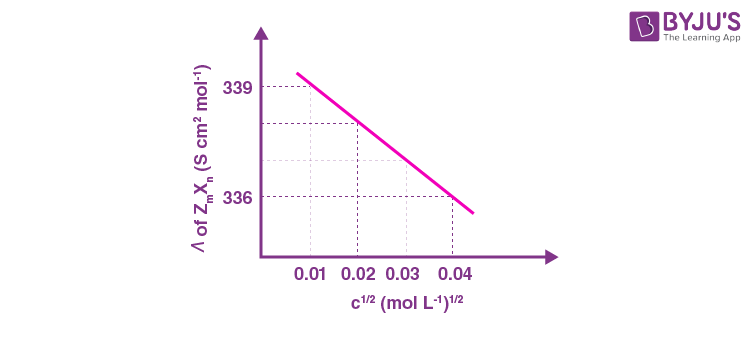Sol.

$$\begin{array}{l}{\lambda _m} = \lambda _m^o – A\sqrt C \end{array}$$

For electrolyte ZmXn and from given curve

$$\begin{array}{l}{\lambda _m}({Z_m}{X_n}) = \lambda _m^o({Z_m}{X_n}) – A\sqrt C \end{array}$$
$$\begin{array}{l} -A=\frac{{336 – 339}}{{0.04 – 0.01}}=-\frac{3}{{0.03}}\end{array}$$

⇒ A = 100

∴ For λm = 336 S cm2 mol-1

$$\begin{array}{l} \Rightarrow 336 = \lambda _m^o({Z_m}{X_n}) – 100 \times 0.04\end{array}$$
$$\begin{array}{l}\lambda _m^o = 336 + 4 = 340\ {\rm{ S c}}{{\rm{m}}^2}{\rm{ mo}}{{\rm{l}}^{ – 1}}\end{array}$$

ZmXn → mZn+ + nXm-

∴ 50m + 80n = 340

⇒ 5m + 8n = 34 …(i)

UmYp → mUp+ + pYm-

$$\begin{array}{l} \therefore 25m + 100p =\lambda _m^o({U_m}{Y_p}) = 250\end{array}$$

⇒ m + 4p = 10 …(ii)

∴ 100m + 80n = 440

⇒ 5m + 4n = 22 …(iii)

From equation (i) and (iii)

n = 3

m = 2

From equation (ii)

p = 2

∴ m + n + p = 2 + 3 + 2 = 7

4. The reaction of Xe and O2F2 gives a Xe compound P. The number of moles of HF produced by the complete hydrolysis of 1 mol of P is _______.

Sol.

$$\begin{array}{l}Xe{\rm{ }} + {\rm{ }}2{O_2}{F_2}{\rm{ }} \to {\rm{ }}\mathop {Xe{F_4}}\limits_{(P)} {\rm{ }} + {\rm{ }}2{O_2}\end{array}$$
$$\begin{array}{l}\underset{(P)}{6XeF_4}+12H_2O\longrightarrow 4Xe + 2XeO_3+24HF + 3O_2\end{array}$$

Number of moles of HF formed by complete hydrolysis of 1 mole of (P) = 4

5. Thermal decomposition of AgNO3 produces two paramagnetic gases. The total number of electrons present in the antibonding molecular orbitals of the gas that has the higher number of unpaired electrons is _______.

Sol.

$$\begin{array}{l}2AgNO_3(s)\overset{\Delta}{\longrightarrow}2Ag(s)+2NO_2(g)+O_2(g)\end{array}$$

Both the NO2 and O2 gases are paramagnetic. NO2(g) has 1 unpaired electron and O2(g) has 2 unpaired electrons. According to MOT, electronic configuration of O2 is

$$\begin{array}{l}\sigma _{1\;s}^2\;\sigma *_{1\;s}^2\;\sigma _{{\rm{2}}s}^{\rm{2}}\;\sigma *_{2s}^2\;\sigma _{2{p_{\rm{2}}}}^2\;\pi _{2{p_x}}^2\; = \;\pi _{_{2{p_y}}}^2\;\pi *_{2{p_x}}^1\, = \pi *_{2{p_y}}^1\end{array}$$

Total number of electrons present in antibonding molecular orbitals = 6

6. The number of isomeric tetraenes (NOT containing sp-hybridized carbon atoms) that can be formed from the following reaction sequence is ________.Sol. :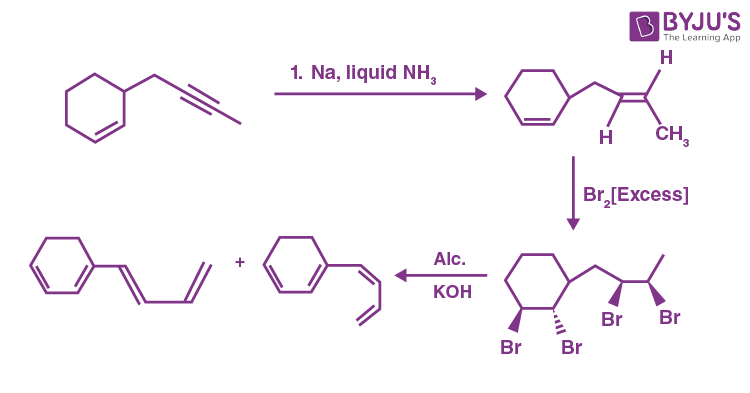7. The number of –CH2– (methylene) groups in the product formed from the following reaction sequence
is ________.Sol.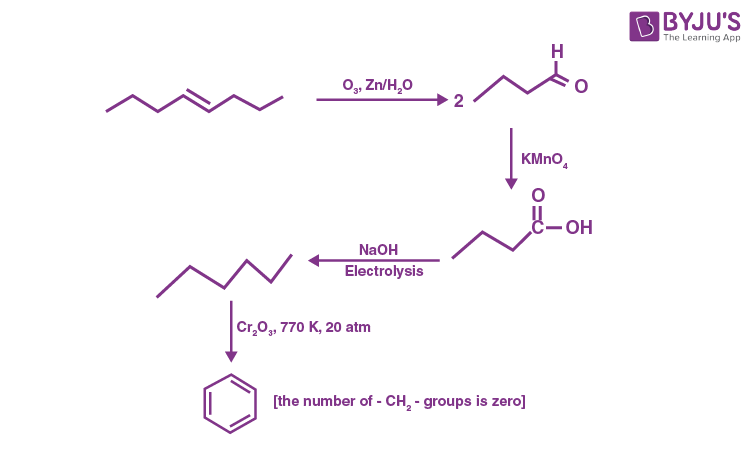8. The total number of chiral molecules formed from one molecule of P on complete ozonolysis (O3, Zn/H2O) is ________.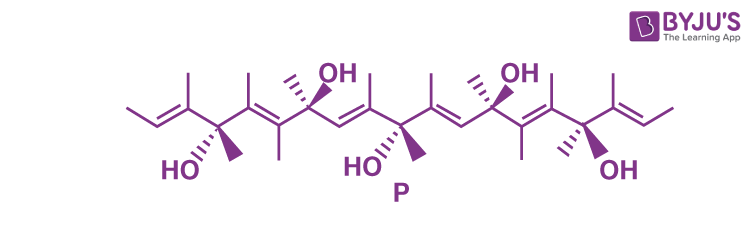Sol.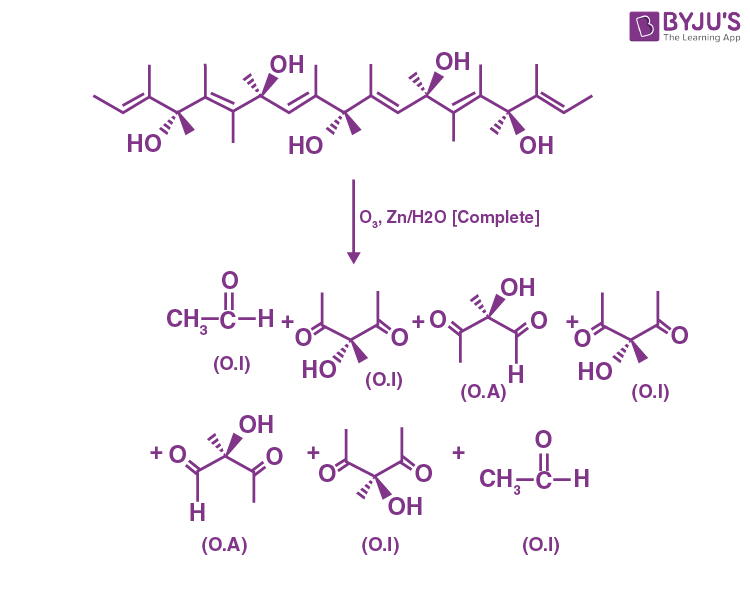∴ Two chiral molecules are obtained.

SECTION – 2 (Maximum marks : 24)

This section contains SIX (06) questions.

Each question has FOUR options (A), (B), (C) and (D). ONE OR MORE THAN ONE of these four option(s) is (are) correct answer(s).

∙ For each question, choose the option(s) corresponding to (all) the correct answer(s).

∙ Answer to each question will be evaluated according to the following marking scheme:

Full Marks : +4 ONLY if (all) the correct option(s) is(are) chosen;

Partial Marks : +3 If all the four options are correct but ONLY three options are chosen;

Partial Marks : + 2 If three or more options are correct but ONLY two options are chosen, both of which are correct;

Partial Marks : +1 If two or more options are correct but ONLY one option is chosen and it is a correct option;

Zero Marks : 0 If none of the options is chosen (i.e. the question is unanswered);

Negative Marks : –2 In all other cases.

9. To check the principle of multiple proportions, a series of pure binary compounds (PmQn) were analyzed and their composition is tabulated below. The correct option(s) is(are)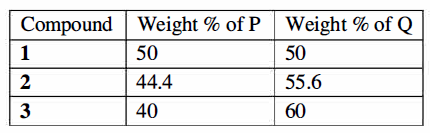(A) If empirical formula of compound 3 is P3Q4, then the empirical formula of compound 2 is P3Q5.

(B) If empirical formula of compound 3 is P3Q2 and atomic weight of element P is 20, then the atomic weight of Q is 45.

(C) If empirical formula of compound 2 is PQ, then the empirical formula of the compound 1 is P5Q4.

(D) If atomic weight of P and Q are 70 and 35, respectively, then the empirical formula of compound 1 is P2Q.

Sol. (A) If empirical formula of 3 is P3Q4, then molecular formula is (P3Q4)n

$$\begin{array}{l} \Rightarrow \frac{{3{M_P}}}{{3{M_P} + 4{M_Q}}} = \frac{{40}}{{100}} = \frac{2}{5}\end{array}$$

15MP = 6MP + 8MQ

9MP = 8MQ

$$\begin{array}{l}\therefore \text{For} P_3Q_5, \text{percentage of P} = \left( {\frac{{3{M_P}}}{{3{M_P} + 5{M_Q}}}} \right) \times 100\end{array}$$
$$\begin{array}{l}=\frac {8M_Q}{23M_Q} \times 100\\ \simeq 34.78~ \% \end{array}$$

(B) If empirical formula of compound 3 is P3Q2, we have

$$\begin{array}{l}\frac{{3{M_P}}}{{3{M_P} + 2{M_Q}}} = \frac{2}{5}\end{array}$$

15MP = 6MP + 4MQ

9MP = 4MQ

If MP = 20

$$\begin{array}{l}{M_Q} = \frac{{180}}{4} = 45\end{array}$$

(C) If empirical formula of 2 is PQ

$$\begin{array}{l} \therefore \frac{{{M_P}}}{{{M_P} + {M_Q}}} = \frac{4}{9} \simeq \frac{{44.44}}{{100}}\end{array}$$

9MP = 5MP + 4MQ

5MP = 4MQ

If empirical formula is assumed as P5Q4,

$$\begin{array}{l}\%\ of\ P = \left( {\frac{{5{M_P}}}{{5{M_P} + 4{M_Q}}}} \right) \times 100 = 50\end{array}$$

Hence P5Q4 is the empirical formula of compound

(D) If empirical formula of I is P2Q, we have

$$\begin{array}{l}\%\ of\ P = \left( {\frac{{2{M_P}}}{{2{M_P} + {M_Q}}}} \right) \times 100 = 50\end{array}$$

4MP = 2MP + MQ

2MP = MQ

Hence, atomic weight of P and Q cannot be 70 and 35 respectively.

Hence (B, C) are correct.

10. The correct option(s) about entropy (S) is(are)

[R = gas constant, F = Faraday constant, T = Temperature]

(A) For the reaction, M(s) + 2H+(aq) → H2(g) + M2+(aq), if

$$\begin{array}{l}\frac{{d{E_{cell}}}}{{dT}} = \frac{R}{F}\end{array}$$
then the entropy change of the reaction is R (assume that entropy and internal energy changes are temperature independent).

(B) The cell reaction, Pt(s) | H2(g, 1bar) | H+(aq, 0.01M) || H+(aq, 0.1M) | H2(g, 1bar) | Pt(s), is an entropy driven process.

(C) For racemization of an optically active compound, ΔS > 0

(D) ΔS > 0, for [Ni(H2O)6]2+ + 3 en → [Ni(en)3]2+ + 6H2O (where en = ethylenediamine).

Sol. (A) M(s) + 2H+(aq) → H2(g) + M2+(aq)

if

$$\begin{array}{l}\frac{{d{E_{cell}}}}{{dT}} = \frac{R}{F}\end{array}$$
$$\begin{array}{l}\Delta S = nF\frac{{dE}}{{dT}}\end{array}$$
=
$$\begin{array}{l}2F\left( {\frac{R}{F}} \right)\end{array}$$
= 2R

(B)

$$\begin{array}{l}{E_{cell}} = \frac{{ – 2.303RT}}{F}\log \frac{{0.01}}{{0.1}}\end{array}$$
$$\begin{array}{l} = \frac{{2.303RT}}{F}\end{array}$$
$$\begin{array}{l}\frac{{d{E_{cell}}}}{{dT}} = \frac{{2.303R}}{F}\end{array}$$
$$\begin{array}{l}\therefore \Delta S = nF\frac{{dE}}{{dT}}\; > \;0\end{array}$$

It is an entropy driven process.

(C) It is correct

During racemisation of optically active compound, disorder increases and hence, entropy increases.

(D) For [Ni(H2O)6]2+ + 3en → [Ni(en)3]+3 + 6H2O,

Entropy increases when bidentate ligands replace monodentate ligands due to increase in the number of molecules on the product side.

Hence, (B, C, D) are correct.

11. The compound(s) which react(s) with NH3 to give boron nitride (BN) is(are)

(A) B

(B) B2H6

(C) B2O3

(D) HBF4

Sol. The compound(s) which react with NH3 to form Boron nitride (BN) is/are:

$$\begin{array}{l}(A)\ \text{Amorphous}\ B + NH_3\xrightarrow[\text{Temperature}]{\text{Very high}}BN + H_2\end{array}$$
$$\begin{array}{l}(B)\ B_2H_6+ \underset{(excess)}{NH_3}\xrightarrow[\text{}]{\text{High Temp.}}(BN)_x\end{array}$$
$$\begin{array}{l}(C)\ B_2O_3+2NH_3\xrightarrow[\text{}]{900^\circ C}2BN + 3H_2O\end{array}$$
$$\begin{array}{l}(D)\ HBF_4 + NH_3 \longrightarrow[NH_4^{\oplus}][BF_4^{\ominus}]\end{array}$$

Hence, (A, B, C) are correct

12. The correct option(s) related to the extraction of iron from its ore in the blast furnace operating in the temperature range 900 – 1500 K is(are)

(A) Limestone is used to remove silicate impurity.

(B) Pig iron obtained from blast furnace contains about 4% carbon.

(C) Coke (C) converts CO2 to CO.

(D) Exhaust gases consist of NO2 and CO.

Sol. (A) Limestone is added to remove silica as impurity.

(B) Pig iron obtained from blast furnace contains 4% carbon and many other impurities (eg. S, P, Si, Mn) in small amount.

(C) Coke (C) converts CO2 in CO.

C + CO2 → 2CO

(D) Exhaust gases consist of CO and CO2.

Hence, (A, B, C) are correct.

13. Considering the following reaction sequence, the correct statement(s) is(are)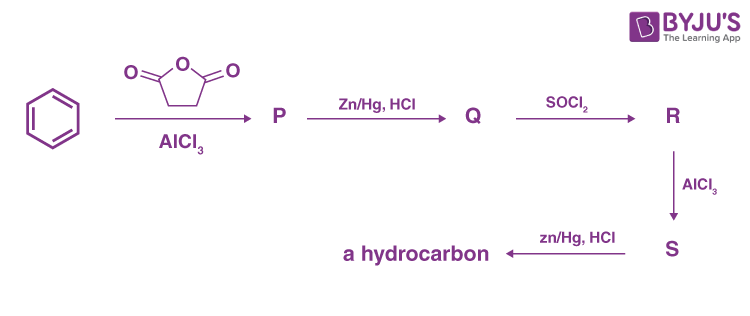(A) Compounds P and Q are carboxylic acids.

(B) Compound S decolorizes bromine water.

(C) Compounds P and S react with hydroxylamine to give the corresponding oximes.

(D) Compound R reacts with dialkylcadmium to give the corresponding tertiary alcohol.

Sol.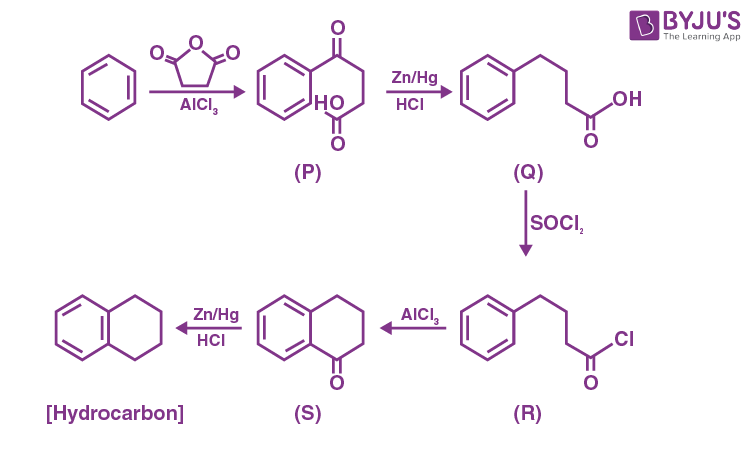Compound (S) can not decolorizes bromine water.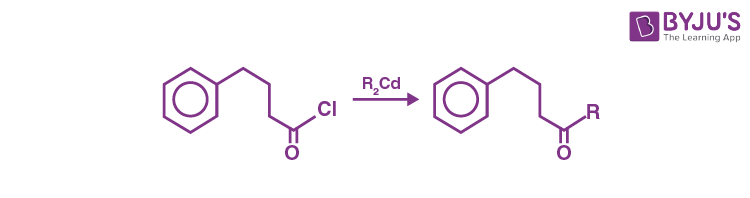R2Cd is a less reactive nucleophile so reaction stops at carbonyl group.

14. Among the following, the correct statement(s) about polymers is(are)

(A) The polymerization of chloroprene gives natural rubber.

(B) Teflon is prepared from tetrafluoroethene by heating it with persulphate catalyst at high pressures.

(C) PVC are thermoplastic polymers.

(D) Ethene at 350-570 K temperature and 1000-2000 atm pressure in the presence of a peroxide initiator yields high density polythene.

Sol. (A) Polymerization of chloroprene gives synthetic rubber

(B) Teflon is manufactured by heating tetrafluoroethene with a free radical or persulphate catalyst at hight pressure.

(C) Polyvinyl chloride (PVC) is a thermoplastic polymer and used in making hand bags, vinyl flooring and water pipes.

(D) Ethene at 350-570 K temperature and 1000-2000 atm pressure in the presence of a peroxide initiator yields low density polythene.

SECTION – 3 (Maximum marks : 12)

∙ This section contains FOUR (04) questions.

∙ Each question has FOUR options (A), (B), (C) and (D). ONLY ONE of these four options is the correct answer.

∙ For each question, choose the option corresponding to the correct answer.

∙ Answer to each question will be evaluated according to the following marking scheme:

Full Marks : +3 If ONLY the correct option is chosen;

Zero Marks : 0 If none of the options is chosen (i.e. the question is unanswered);

Negative Marks : −1 In all other cases.

15. Atom X occupies the fcc lattice sites as well as alternate tetrahedral voids of the same lattice. The packing efficiency (in %) of the resultant solid is closest to

(A) 25

(B) 35

(C) 55

(D) 75

Sol. Atom X occupies FCC lattice sites as well as alternate tetrahedral voids of FCC.

In FCC, tetrahedral voids are 8 (in a unit cell)

Hence

$$\begin{array}{l} \text{Atom X in a unit cell \(FCC lattice sites$$} = 8 \times \frac{1}{8} + 6 \times \frac{1}{2} = 4\end{array} \)
$$\begin{array}{l} \text{Atom X in a unit cell (in T.V.)} = \frac{1}{2} \times 8 = 4\end{array}$$

Total atom X in one unit cell = 8

For relation between a and r, since T.V. forms at ¼ th of body diagonal,

$$\begin{array}{l}\frac{{a\sqrt 3 }}{4} = 2{\mathop{\rm r}\nolimits} \end{array}$$
$$\begin{array}{l} a =\frac{{8{\mathop{\rm r}\nolimits} }}{{\sqrt 3 }}\end{array}$$
$$\begin{array}{l}\text{Packing efficiency}= \frac{{8 \times \frac{4}{3}\pi {r^3}}}{a^3}\times 100\end{array}$$
$$\begin{array}{l}=\frac{8\times \frac{4}{3}\pi r^3}{\left ( \frac{8r}{\sqrt{3}} \right )^3}\times 100\simeq 35\%\end{array}$$

16. The reaction of HClO3 with HCl gives a paramagnetic gas, which upon reaction with O3 produces

(A) Cl2O

(B) ClO2

(C) Cl2O6

(D) Cl2O7

Sol. HClO3 reacts with HCl according to the following equation,

$$\begin{array}{l}2HClO_3 + 2HCl \longrightarrow 2ClO_2+Cl_2+2H_2O\end{array}$$

ClO2 molecule is paramagnetic, as it contains odd number of electrons.

$$\begin{array}{l}2ClO_2+2O_3 \longrightarrow Cl_2O_6+2O_2\end{array}$$

17. The reaction of Pb(NO3)2 and NaCl in water produces a precipitate that dissolves upon the addition of HCl of appropriate concentration. The dissolution of the precipitate is due to the formation of

(A) PbCl2

(B) PbCl4

(C) [PbCl4]2–

(D) [PbCl6]2–

Sol. Pb2+ on reaction with Cl, produces white precipitate of PbCl2

$$\begin{array}{l}Pb^{2+}+2Cl^- \longrightarrow \underset{White}{PbCl_2}\downarrow\end{array}$$

This precipitate is soluble in concentrated hydrochloric acid due to formation of tetrachloroplumbate (II) ion

$$\begin{array}{l}{PbCl_2}\downarrow+2Cl^- \longrightarrow [PbCl_4]^{2-}\end{array}$$

18. Treatment of D-glucose with aqueous NaOH results in a mixture of monosaccharides, which are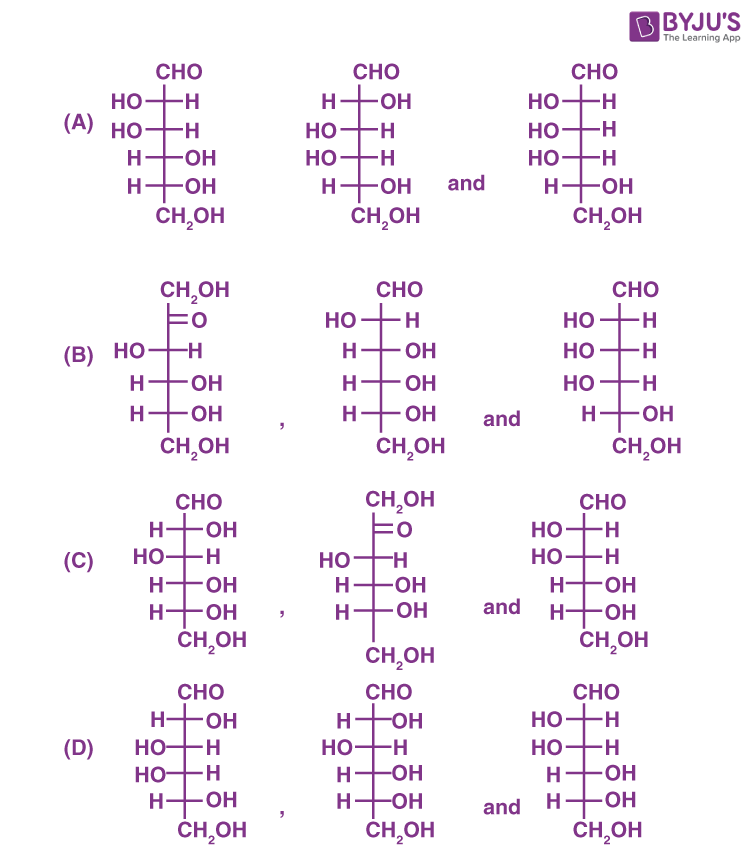Sol.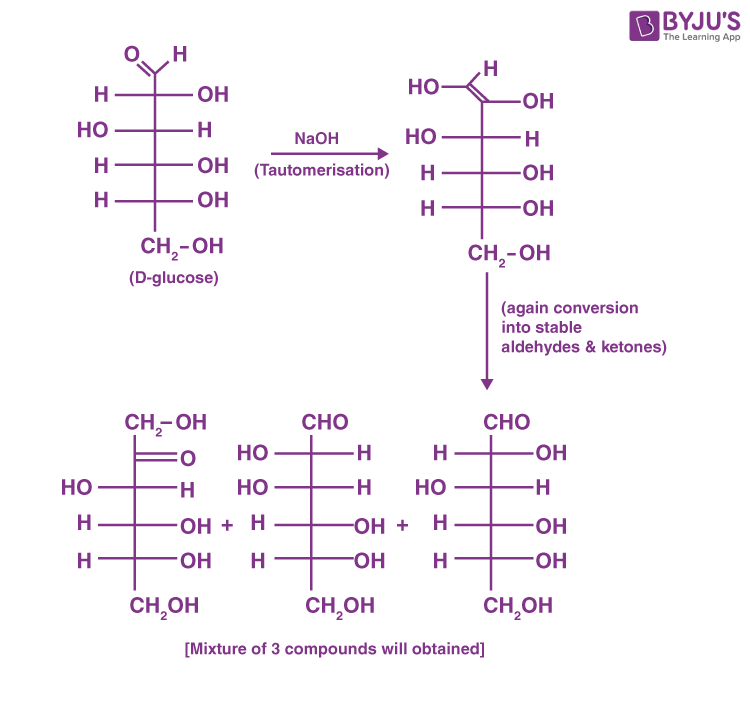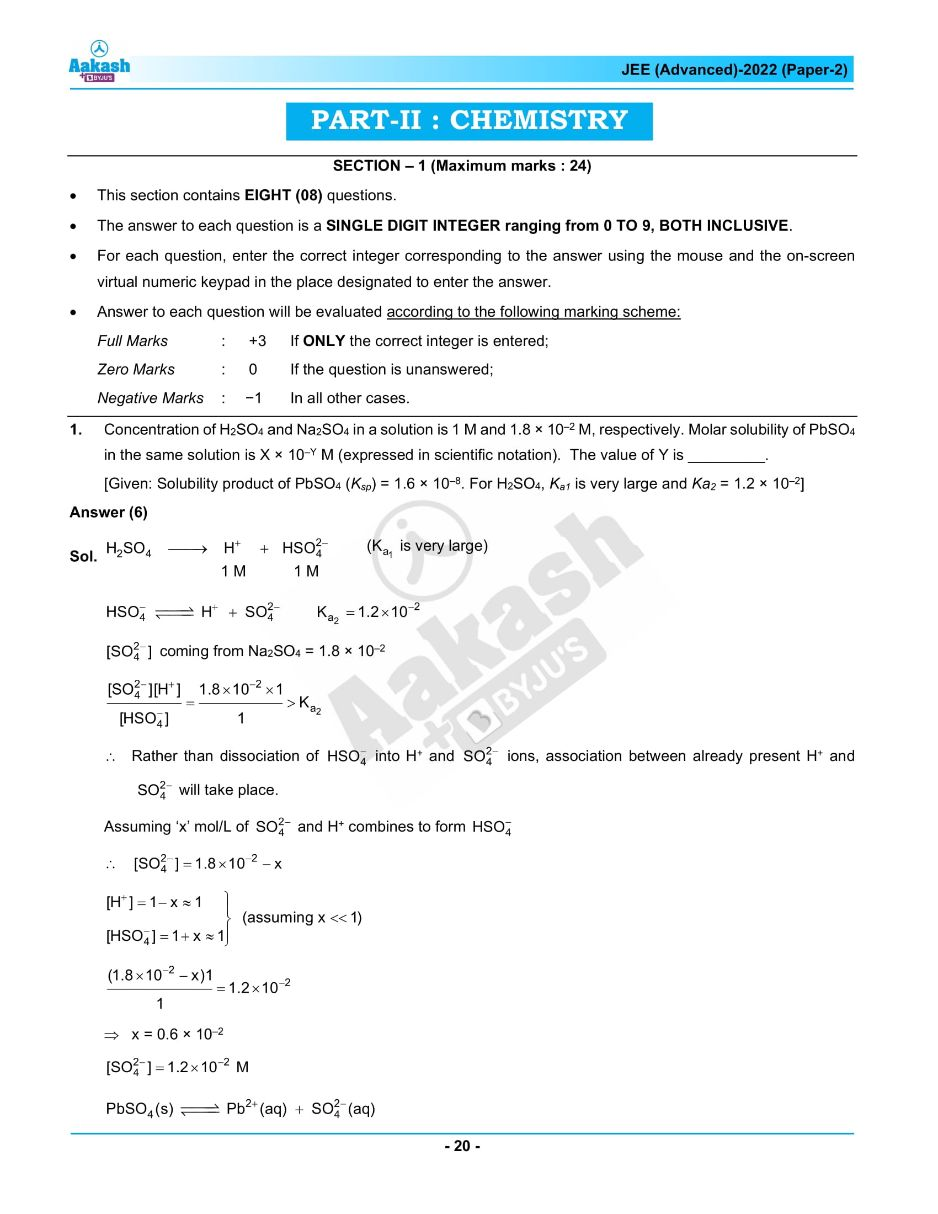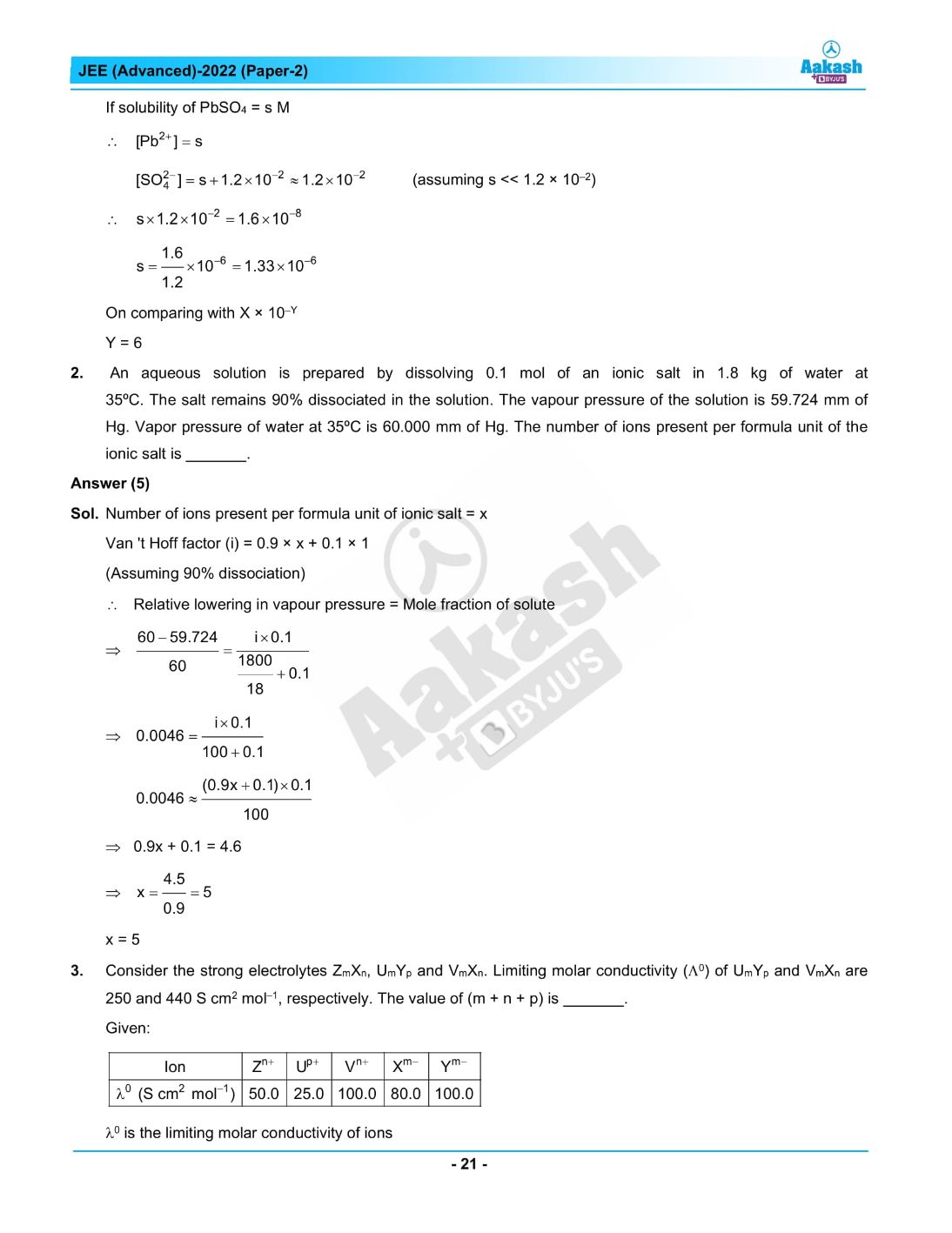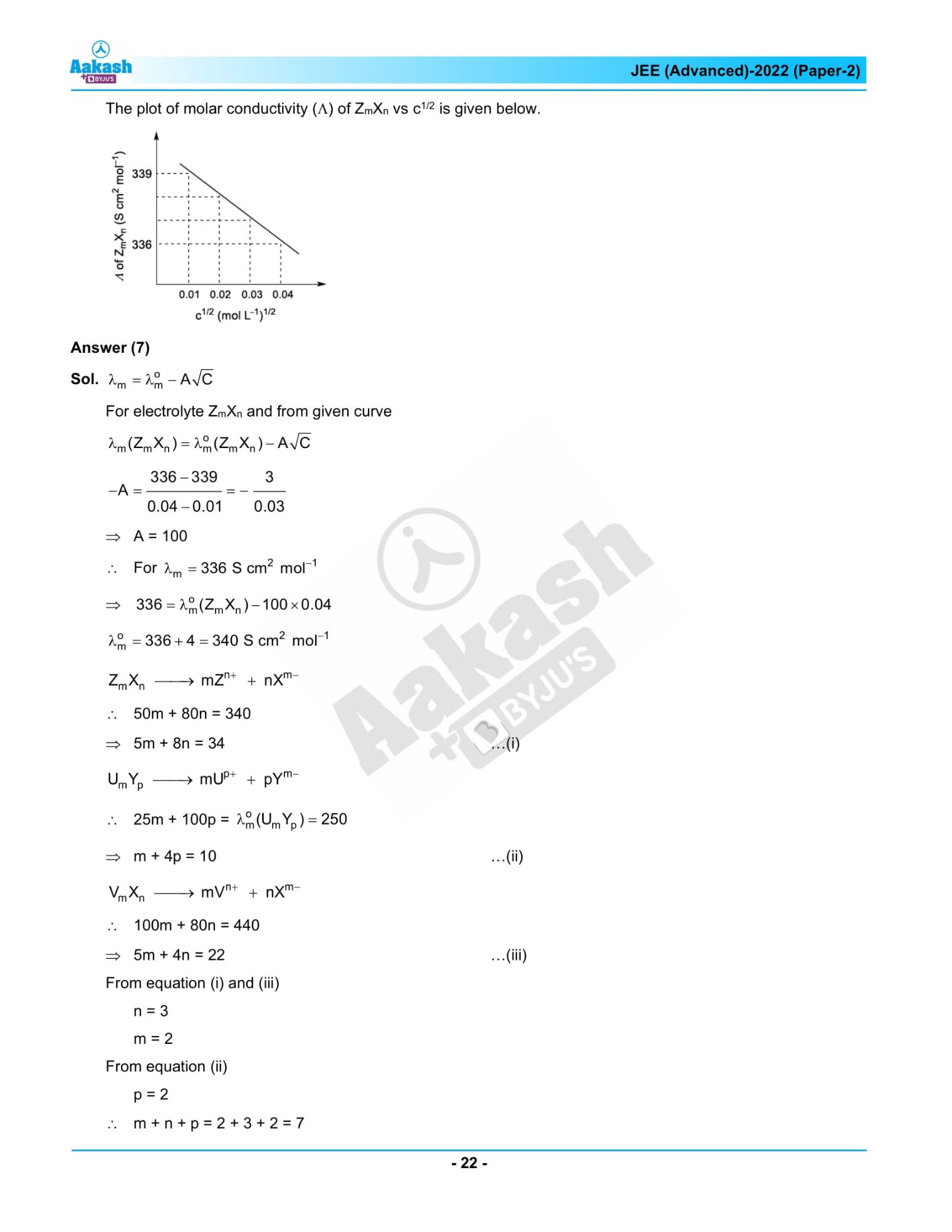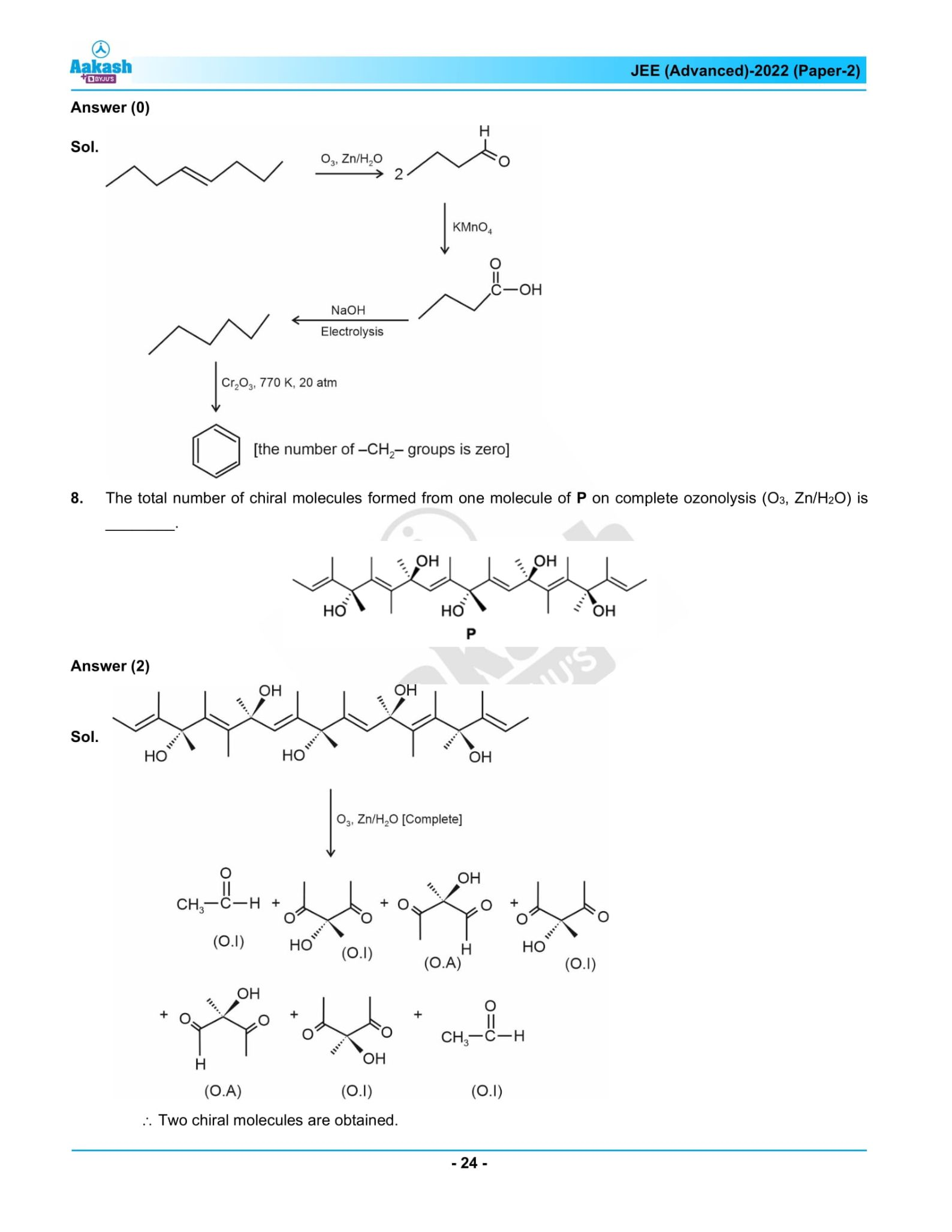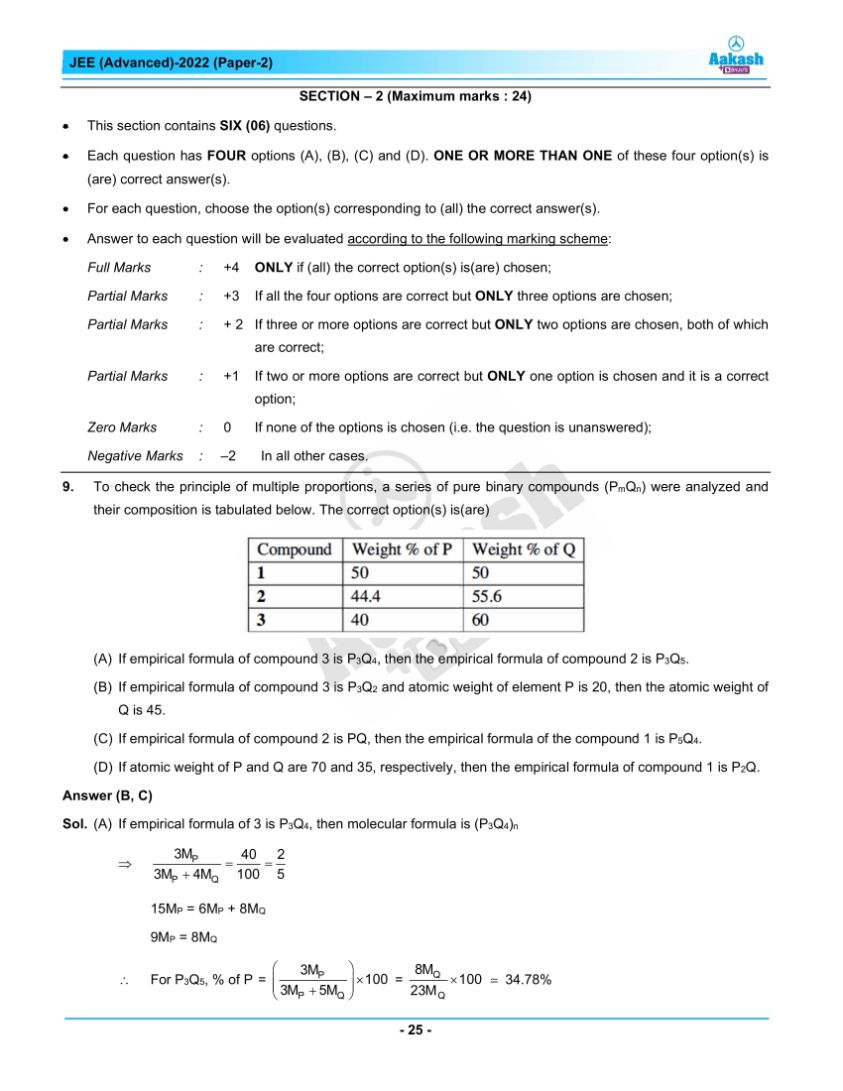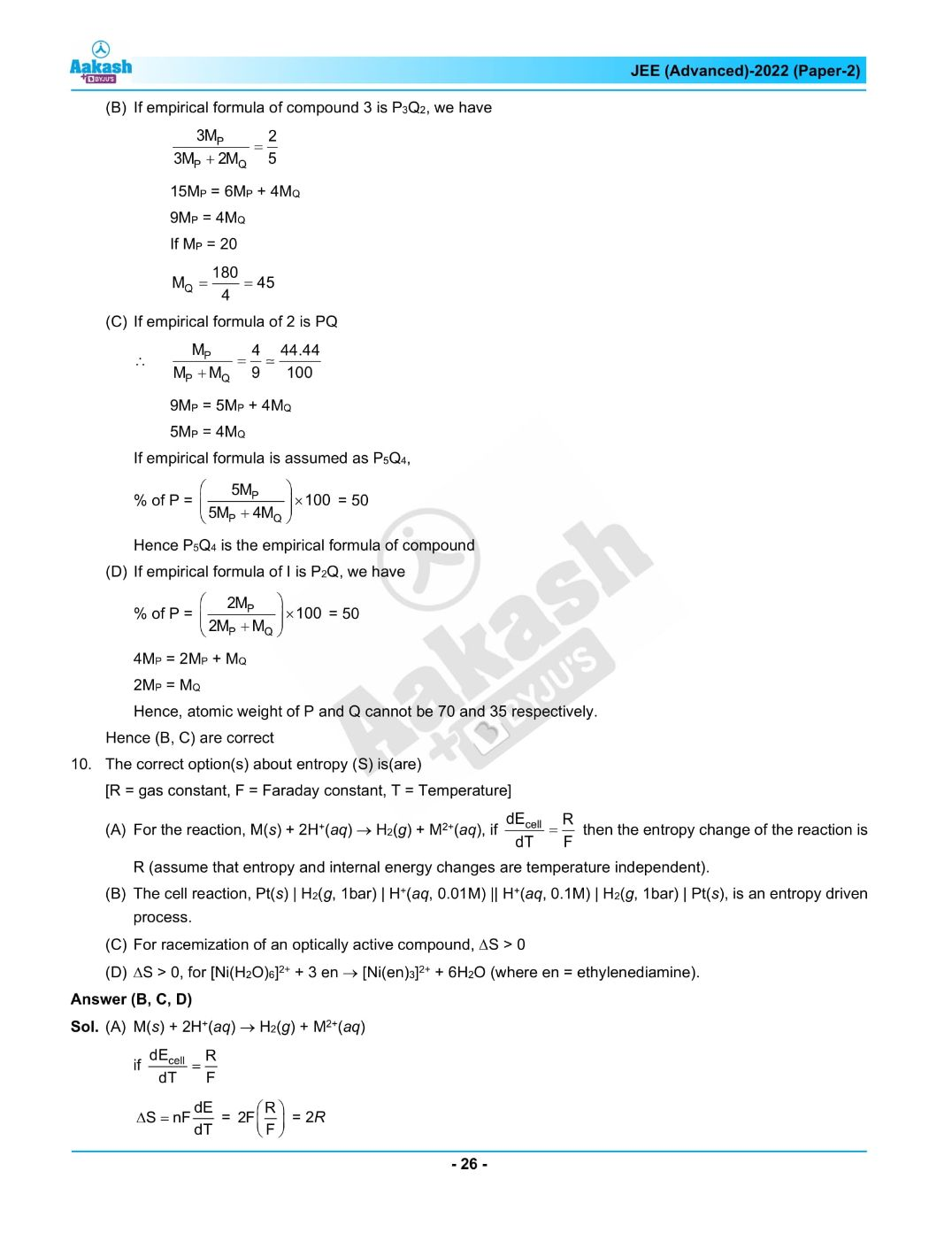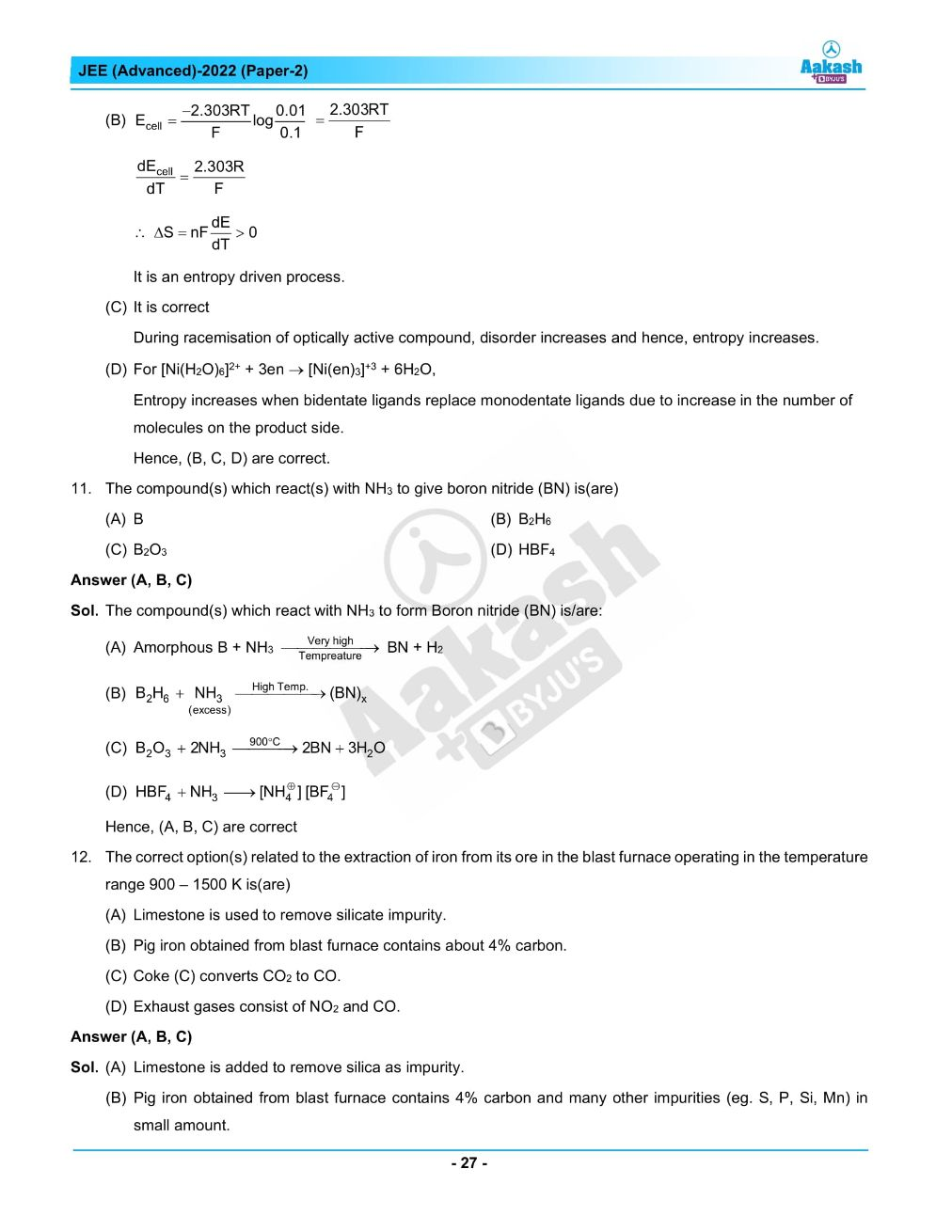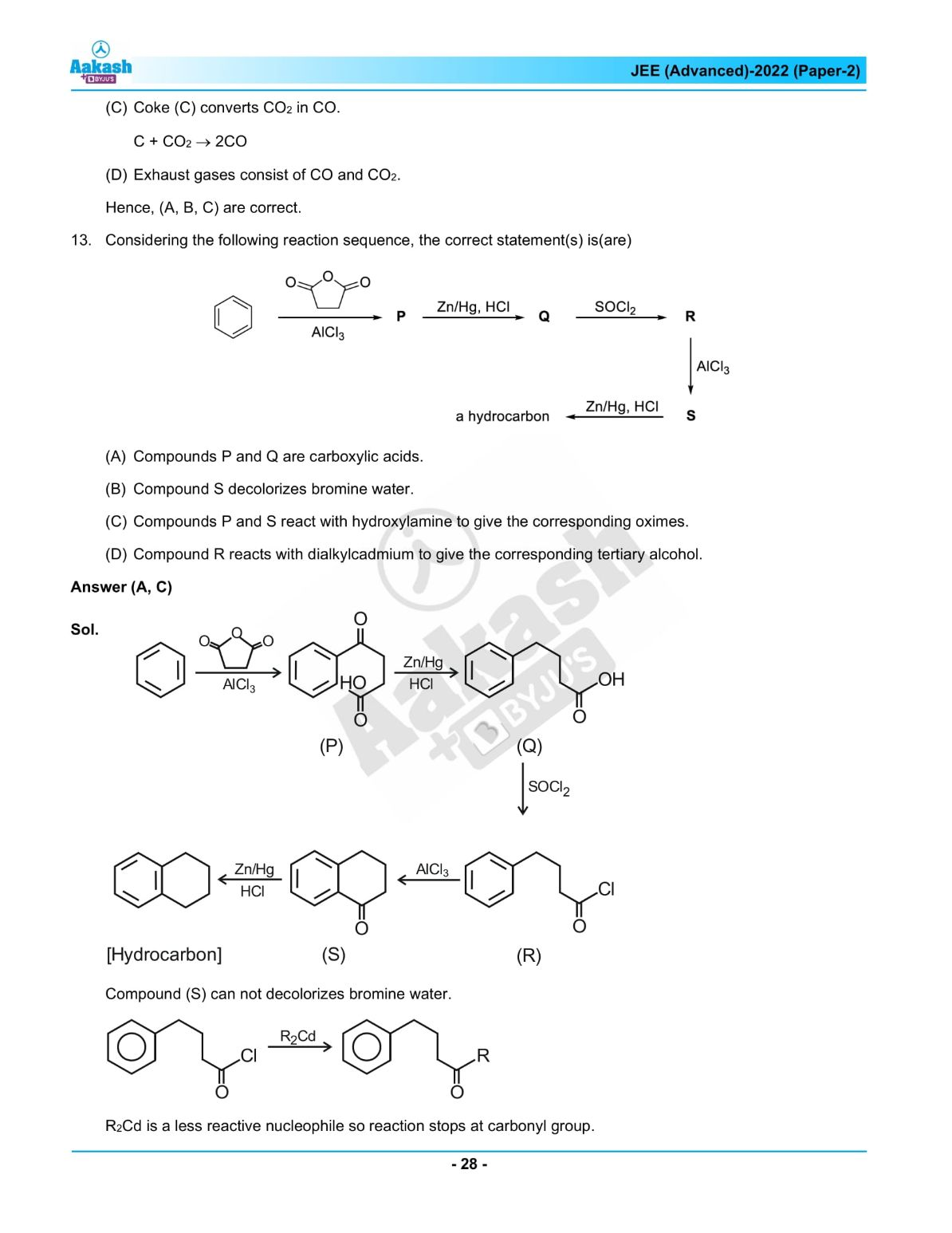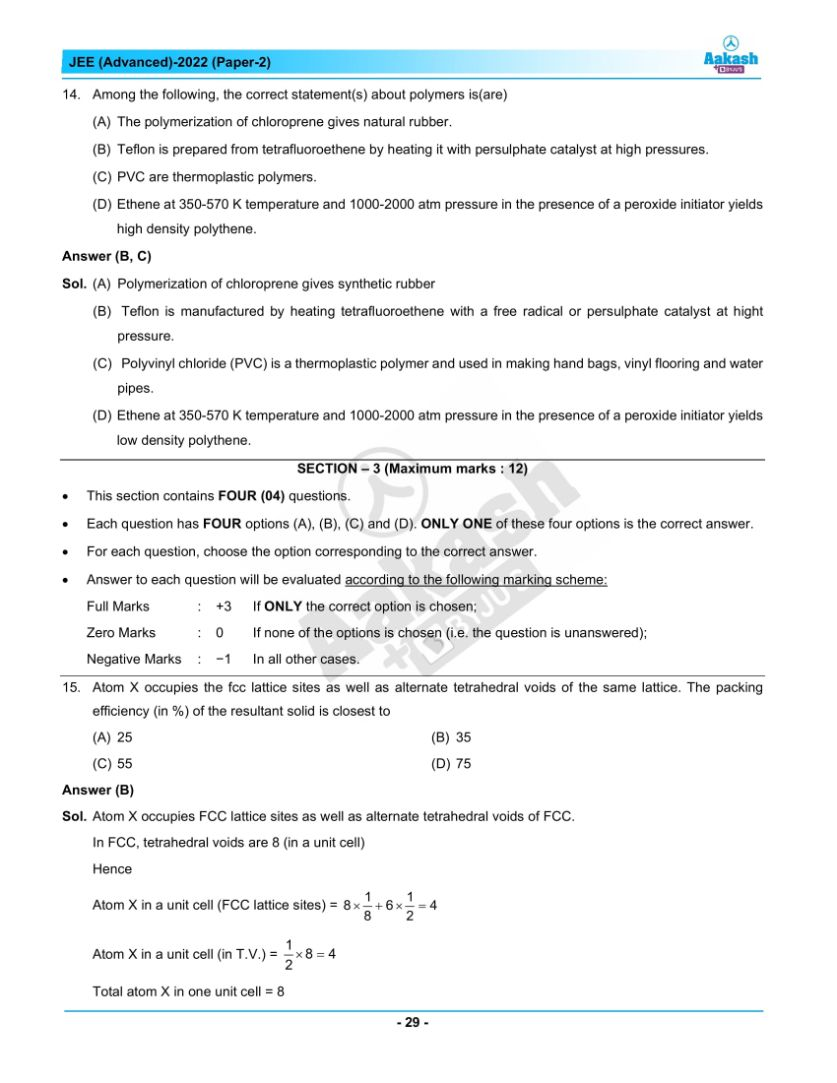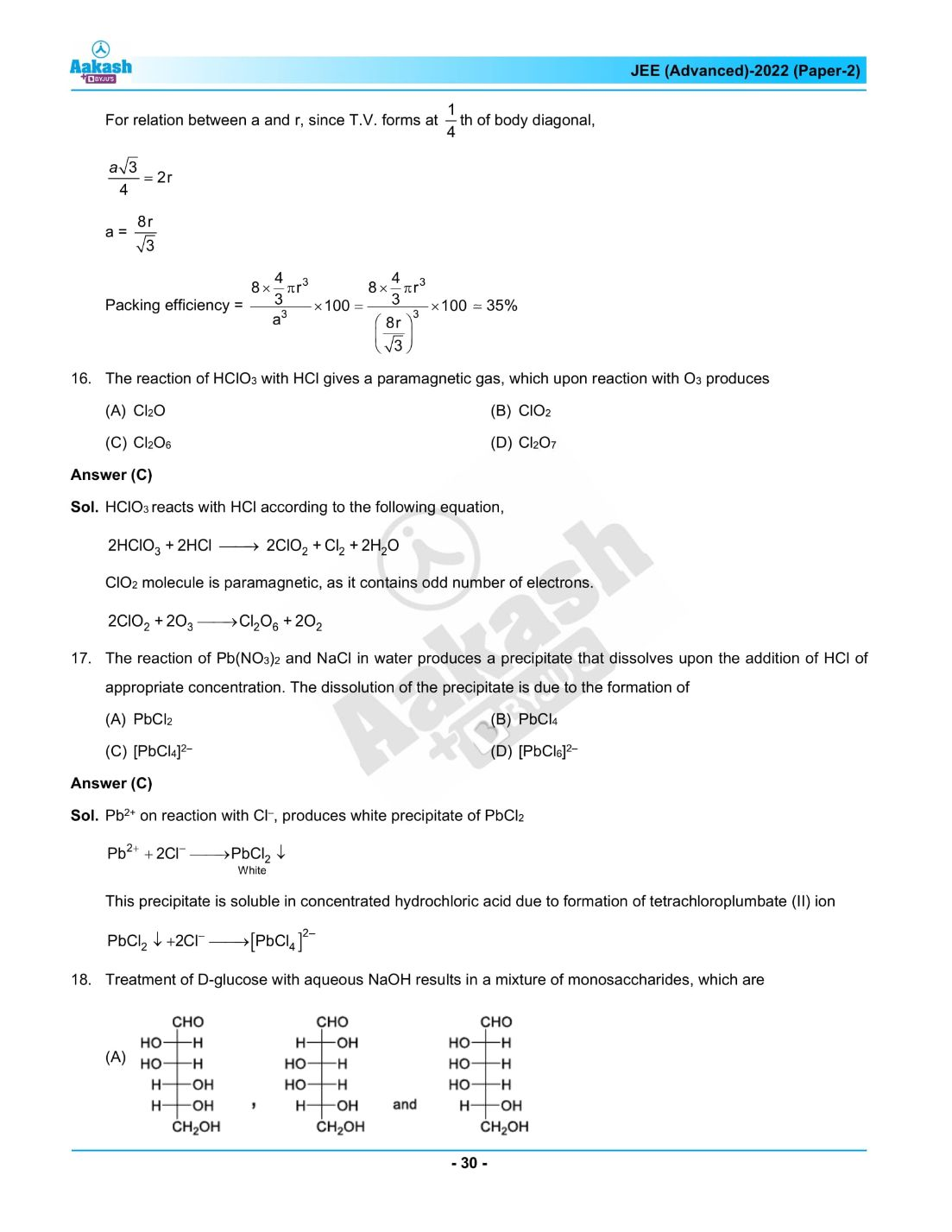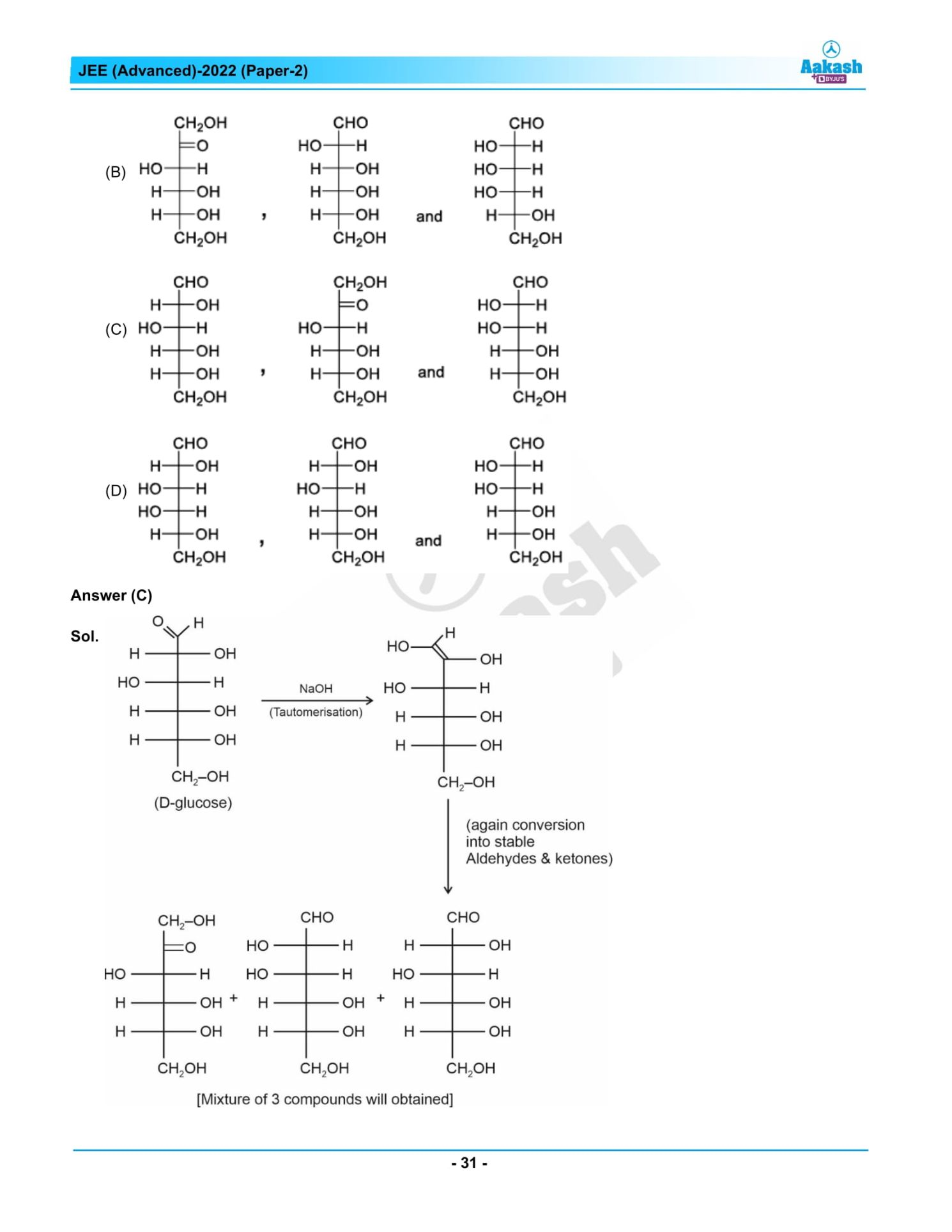## JEE Advanced 2022 Paper 2 – Live Analysis and Solutions## Frequently Asked Questions – FAQs

Q1

### Was there any negative marking in JEE Advanced 2022 Chemistry question paper 2?

Yes. Negative marking was there for MCQs and MSQs in JEE Advanced 2022 Chemistry question paper 2.
Q2

### What was the difficulty level of JEE Advanced 2022 Chemistry paper 2?

On a scale of 3, we can define the overall difficulty level of JEE Advanced 2022 Chemistry paper 2 as 2.17.
Q3

### Was any question asked from P-block elements in JEE Advanced 2022 Chemistry question paper 2?

Yes, two questions were asked from P-block elements in JEE Advanced 2022 Chemistry question paper 2, one from the class 11 syllabus and another from the class 12 syllabus.
Q4

### What was the distribution of questions in the JEE Advanced question paper 2022 chemistry paper 2?

Chemistry part of the JEE Advanced 2022 question paper 2 had 11 medium and 2 easy, and
5 difficult questions.
Q5

### What are the chapters from which easy questions appeared in the JEE Advanced 2022 Chemistry paper 2?

Easy questions appeared in the JEE Advanced 2022 Chemistry paper 2 from the P-block elements and Polymers.